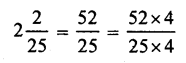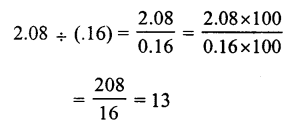# RS Aggarwal Class 7 Solutions Chapter 3 Decimals Ex 3E

In this chapter, we provide RS Aggarwal Solutions for Class 7 Chapter 3 Decimals Ex 3E for English medium students, Which will very helpful for every student in their exams. Students can download the latest RS Aggarwal Solutions for Class 7 Chapter 3 Decimals Ex 3E Maths pdf, free RS Aggarwal Solutions Class 7 Chapter 3 Decimals Ex 3E Maths book pdf download. Now you will get step by step solution to each question.

### RS Aggarwal Solutions for Class 7 Chapter 3 Decimals Ex 3E Download PDF

Question 1.
Solution:
(b)Question 2.
Solution:
(c)Question 3.
Solution:
(b)= 208100 = 2.08

Question 4.
Solution:Question 5.
Solution:
(b) 70g = 701000 = 0.07 kg

Question 6.
Solution:
(c) 5 kg 6 g = 561000 kg = 5.006 kg

Question 7.
Solution:
(c) 2 km 5 m = 251000 km = 2.005 km

Question 8.
Solution:
(c)
1.007 – 0.7 = 1.007 – 0.700 = 0.307

Question 9.
Solution:
(b)
0.1 – 0.03 = 0.10 – 0.03 = 0.07

Question 10.
Solution:
(c)
3.5 – 3.07 = 3.50 – 3.07 = 0.43

Question 11.
Solution:
(c)
0.23 x 0.3 = 0.069

Question 12.
Solution:
(b)
0.02 x 30 = .60 = .6

Question 13.
Solution:
(b)
0.25 x 0.8 = 0.200 = 0.2

Question 14.
Solution:
(c)
0.4 x 0.4 x 0.4 = 0.064

Question 15.
Solution:
(b)
1.1 x .1 x .01 = .0011

Question 16.
Solution:
(a)Question 17.
Solution:
(b)
1.02 ÷ 6 = 1.026 = 0.17

Question 18.
Solution:Question 19.
Solution:
(b)Question 20.
Solution:
(a)Question 21.
Solution:
(c)All Chapter RS Aggarwal Solutions For Class 7 Maths

—————————————————————————–

All Subject NCERT Exemplar Problems Solutions For Class 7

All Subject NCERT Solutions For Class 7

*************************************************

I think you got complete solutions for this chapter. If You have any queries regarding this chapter, please comment on the below section our subject teacher will answer you. We tried our best to give complete solutions so you got good marks in your exam.

If these solutions have helped you, you can also share rsaggarwalsolutions.in to your friends.